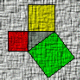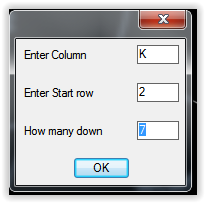# More compact code for Excel link

## Recommended PostsI am trying to write an autolisp program to insert a row of 20 blocks in a drawing, (one over the other), then insert attributes for each from a row in an Excel file. I am using Jeffrey Sanders' "GetCells" function.

The Excel file is "RO-16.xlsx"

Here's what I have done so far:

(defun Addenters ( / cnt yval)

(setq cnt 1)

(setq yval 0)

(setvar "osmode" 0)

(while (

(

(command "insert" "C:\\07509\\BD" (strcat "0," (itoa yval))"1" "1" "04" (getCellsFunction "C:\\07509\\RO-16.xlsx" "ALLOCATED" "K2") )

(setq cnt (1+ cnt))

(setq yval (+ 8 yval))

)

)

)

So the first attribute, "PLC" is read from cell K2 in the Excel file. There are 7 attributes altogether. Is there a more compact way to do this (with a list or array). I want to avoid having a long line (which is answering to the Autocad prompt for block attributes) which looks like: (getCellsFunction "C:\\07509\\RO-16.xlsx" "ALLOCATED" "L2"), (getCellsFunction "C:\\07509\\RO-16.xlsx" "ALLOCATED" "M2") and so on.

##### Share on other sitesYou may want to search a bit more for getxecel.lsp, not sure whats in getcells.lsp but one option is to not use the "K2" but rather a row,column method so you can have a second loop that k2=11,2 or X Y then increment X up to 8 K8. You may want to hack the code a bit more so that (getcellsfunction k2) by locking in the spreadsheet name and workbook early in the code. I noticed in the getxecel.lsp I just looked at that there is a function called getrows (getrows K2 7) start at k2 and get next 7 cells.

had a look at getcells.lsp and you could rewrite pretty easy a x,y style with an extra defun that makes "k2" 11,2 past 26 is a bit of a problem for columns.

```(defun getCellsFunction( cellName / [color=red]fileName sheetName[/color] myXL myBook mySheet myRange cellValue) but you need to ask these two questions next two lines.
;;;--- Get the excel file
[color=red](setq fileName(getfiled "Select Excel File" "" "*" 16)) ; remove from below[/color]
;;;--- Get the sheet name
[color=red](setq sheetName(getstring T "\nName of sheet: ")) ; remove from below[/color]
(setq myXL(vlax-get-or-create-object "Excel.Application"))
```

##### Share on other sitesYou may want to search a bit more for getxecel.lsp, not sure whats in getcells.lsp but one option is to not use the "K2" but rather a row,column method so you can have a second loop that k2=11,2 or X Y then increment X up to 8 K8. You may want to hack the code a bit more so that (getcellsfunction k2) by locking in the spreadsheet name and workbook early in the code. I noticed in the getxecel.lsp I just looked at that there is a function called getrows (getrows K2 7) start at k2 and get next 7 cells.

had a look at getcells.lsp and you could rewrite pretty easy a x,y style with an extra defun that makes "k2" 11,2 past 26 is a bit of a problem for columns.

```(defun getCellsFunction( cellName / [color=red]fileName sheetName[/color] myXL myBook mySheet myRange cellValue) but you need to ask these two questions next two lines.
;;;--- Get the excel file
[color=red](setq fileName(getfiled "Select Excel File" "" "*" 16)) ; remove from below[/color]
;;;--- Get the sheet name
[color=red](setq sheetName(getstring T "\nName of sheet: ")) ; remove from below[/color]
(setq myXL(vlax-get-or-create-object "Excel.Application"))
```

I don't follow - should I remove the code in red from "getcells.lsp"?

##### Share on other sitesWhat BIGAL is telling you is that in the getcells.lsp the function can return a

list of cells (not only a single cell.

```(defun getCells (fileName sheetName cellName / xl workbook sheet range cellValue)
(setq xl(vlax-get-or-create-object "Excel.Application"))
(vla-put-visible xl :vlax-false)
(setq workbook (vl-catch-all-apply 'vla-open (list (vlax-get-property xl "WorkBooks") fileName)))
(setq sheet (vl-catch-all-apply 'vlax-get-property (list (vlax-get-property workbook "Sheets") "Item" sheetName)))
(vlax-invoke-method sheet "Activate")
(setq range (vlax-get-property (vlax-get-property sheet 'Cells) "Range" cellName))
(setq cellValue(vlax-variant-value (vlax-get-property range 'Value2)))
(vl-catch-all-apply 'vlax-invoke-method (list workbook "Close"))
(vl-catch-all-apply 'vlax-invoke-method (list xl "Quit"))
(if (not (vlax-object-released-p range))(progn(vlax-release-object range)(setq range nil)))
(if (not (vlax-object-released-p sheet))(progn(vlax-release-object sheet)(setq sheet nil)))
(if (not (vlax-object-released-p workbook))(progn(vlax-release-object workbook)(setq workbook nil)))
(if (not (vlax-object-released-p xl))(progn(vlax-release-object xl)(setq xl nil)))
(if(= 'safearray (type cellValue))
(progn
(setq tempCellValue(vlax-safearray->list cellValue))
(setq cellValue(list))
(if(= (length tempCellValue) 1)
(progn
(foreach a tempCellValue
(if(= (type a) 'LIST)
(progn
(foreach b a
(if(= (type b) 'LIST)
(setq cellValue(append cellValue (list (vlax-variant-value (car b)))))
(setq cellValue(append cellValue (list (vlax-variant-value b))))
)
)
)
(setq cellValue(append cellValue (list (vlax-variant-value a))))
)
)
)
(progn
(foreach a tempCellValue
(setq tmpList(list))
(foreach b a
(setq tmp(vlax-variant-value b))
(setq tmpList(append tmpList (list tmp)))
)
(setq cellValue(append cellValue tmpList))
)
)
)
)
)
cellValue
)

(defun c:test ()
(setq fname "c:\\users\\ymg\\documents\\testgetcell.xlsx"
shname "sheet1"
range  "B10:G10"
)
(setq lst (getcells fname shname range))
)
```

You would obtain in a list all the value from B10 to G10

##### Share on other sitesOk, thanks for clarifying that

##### Share on other sitesI was trying to say you can declare the filename and sheet as local variable so when getting a cell your code is only (getcell cellreq) ie (setq cellreq "k2")

I had some more questions are you reading multiple blocks from the one spreadsheet, if so then the code should auto search the excel based on the block name you give, look at creating a series of defuns so you can do multiple,

```(setq row (getint "\nStart row number"))
(setq x (getint "\nhow many rows"))
(setq col (getstring "row name"))

(repeat x
(getcell (strcat col (rtos row 2 0)))
(setq row (+ row 1))
)
```

##### Share on other sitesOk, that should shorten my code a lot. thanks.

##### Share on other sitesAnother go

```(if (not AH:getval3) (load "Getvals"))
(ah:getval3 "Enter Column " 5 4 "Enter Start row " 5 4 "How many down" 5 4)
; val1 val2 val3 these are returned as strings
(setq col val1)
(setq row val2)
(setq down (atoi val3))

(repeat down
(setq cell (strcat col row))
(setq row (rtos (+ (atoi row) 1) 2 0))
(princ cell)
;(getcell here)
)
```

GETVALS.lsp

##### Share on other sitesAnother go

```(if (not AH:getval3) (load "Getvals"))
(ah:getval3 "Enter Column " 5 4 "Enter Start row " 5 4 "How many down" 5 4)
; val1 val2 val3 these are returned as strings
(setq col val1)
(setq row val2)
(setq down (atoi val3))

(repeat down
(setq cell (strcat col row))
(setq row (rtos (+ (atoi row) 1) 2 0))
(princ cell)
;(getcell here)
)
```

[ATTACH]57007[/ATTACH]

Just getting back to this.

I was wondering how you got the messagebox to show. Is there some VB code?

Anyway, I ran this with values "C", "2" and "5" and I got this output:

C2C3C4C5C6"C6"

how can I get it to show the cells' contents?

##### Share on other sitesDown load the Getvals link its a auto DCL creator, you need to use the getcell function still it just goes and gets mutliple rows for you.## Join the conversation

You can post now and register later. If you have an account, sign in now to post with your account.
Note: Your post will require moderator approval before it will be visible.×   Pasted as rich text.   Paste as plain text instead

Only 75 emoji are allowed.